• 图像模糊处理的实现 包括有：均值模糊，高斯模糊，运动模糊 一.均值模糊 # 图像模糊处理 # 均值模糊 box blur import cv2 import numpy as np import matplotlib.pyplot as plt if __name__ == "__main__": image...
图像模糊处理的实现
包括有：均值模糊，高斯模糊，运动模糊
实质为使用滤波器算子对图像每个像素点进行卷积处理，改变像素值，实现图像的模糊化。
一.均值模糊
核心为使用均值滤波器。
# 图像模糊处理
# 均值模糊 box blur

import cv2
import numpy as np
import matplotlib.pyplot as plt

if __name__ == "__main__":
image = cv2.imread('Lena.jpg')

image = cv2.cvtColor(image, cv2.COLOR_BGR2GRAY)

# 此为均值模糊
# （30,1）为一维卷积核，指在x，y方向偏移多少位
dst1 = cv2.blur(image, (30, 1))

# 此为中值模糊，常用于去除椒盐噪声
dst2 = cv2.medianBlur(image, 15)

# 自定义卷积核，执行模糊操作，也可定义执行锐化操作
kernel = np.ones([5, 5], np.float32)/25
dst3 = cv2.filter2D(image, -1, kernel=kernel)

plt.subplot(2, 2, 1)
plt.imshow(image)
plt.axis('off')
plt.title('Offical')

plt.subplot(2, 2, 2)
plt.imshow(dst1)
plt.axis('off')
plt.title('Box blur')

plt.subplot(2, 2, 3)
plt.imshow(dst2)
plt.axis('off')
plt.title('median blur')

plt.subplot(2, 2, 4)
plt.imshow(dst3)
plt.axis('off')
plt.title('defined blur')

plt.show()


效果展示：实际上这里使用了均值滤波器、中值滤波器和一个自定义卷积核的滤波器来比较效果。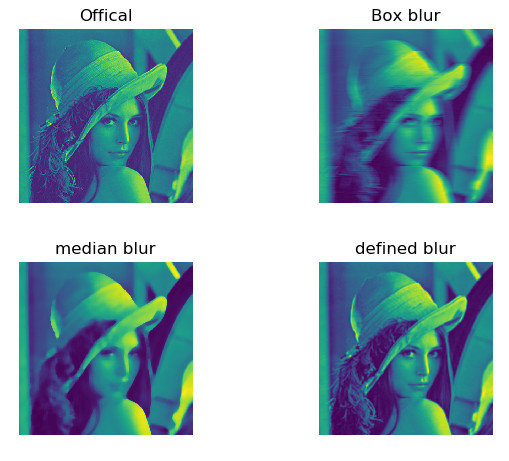二.高斯模糊
# 图像模糊处理
# 高斯模糊 gaussian blur
# 使用自编写高斯噪声及自编写高斯模糊函数与自带高斯函数作效果对比

import cv2
import numpy as np
import matplotlib.pyplot as plt

def clamp(pv):
if pv > 255:
return 255
if pv < 0:
return 0
else:
return pv

def gaussian_noise(image):        # 加高斯噪声
h, w, c = image.shape
for row in range(h):
for col in range(w):
s = np.random.normal(0, 20, 3)
b = image[row, col, 0]   # blue
g = image[row, col, 1]   # green
r = image[row, col, 2]   # red
image[row, col, 0] = clamp(b + s)
image[row, col, 1] = clamp(g + s)
image[row, col, 2] = clamp(r + s)
dst = cv2.GaussianBlur(image, (15, 15), 0)  # 高斯模糊
return dst, image

if __name__ == "__main__":
src = cv2.imread('Lena.jpg')

plt.subplot(2, 2, 1)
plt.imshow(src)
plt.axis('off')
plt.title('Offical')

output, noise = gaussian_noise(src)
cvdst = cv2.GaussianBlur(src, (15, 15), 0)   # 高斯模糊

plt.subplot(2, 2, 2)
plt.imshow(noise)
plt.axis('off')
plt.title('Gaussian Noise')

plt.subplot(2, 2, 3)
plt.imshow(output)
plt.axis('off')
plt.title('Gaussian Blur')

plt.subplot(2, 2, 4)
plt.imshow(cvdst)
plt.axis('off')
plt.title('defined blur by opencv')

plt.show()


结果展示：这里使用了自编写的高斯滤波器和opencv的高斯滤波器来对比效果。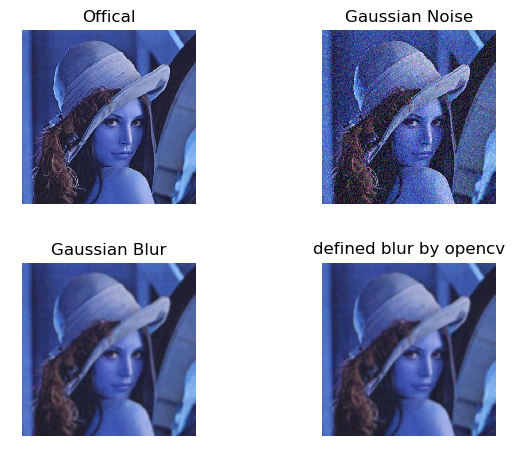三.运动模糊
使用自定义的卷积核进行卷积计算，实现运动模糊效果。
# 图像模糊处理
# 运动模糊，亦称动态模糊，motion blur
# 运动模糊：由于相机和物体之间的相对运动造成的模糊

import numpy as np
import cv2
import matplotlib.pyplot as plt

def motion_blur(image, degree=12, angle=45):

image = np.array(image)
# 这里生成任意角度的运动模糊kernel的矩阵， degree越大，模糊程度越高
M = cv2.getRotationMatrix2D((degree / 2, degree / 2), angle, 1)
motion_blur_kernel = np.diag(np.ones(degree))
motion_blur_kernel = cv2.warpAffine(motion_blur_kernel, M, (degree, degree))
motion_blur_kernel = motion_blur_kernel / degree
blurred = cv2.filter2D(image, -1, motion_blur_kernel)
# convert to uint8
cv2.normalize(blurred, blurred, 0, 255, cv2.NORM_MINMAX)
blurred = np.array(blurred, dtype=np.uint8)
return blurred

if __name__ == "__main__":

img = cv2.imread('Lena.jpg')
dst = motion_blur(img)

plt.subplot(1, 2, 1)
plt.imshow(img)
plt.axis('off')
plt.title('Offical')

plt.subplot(1, 2, 2)
plt.imshow(dst)
plt.axis('off')
plt.title('Motion blur')

plt.show()


结果展示：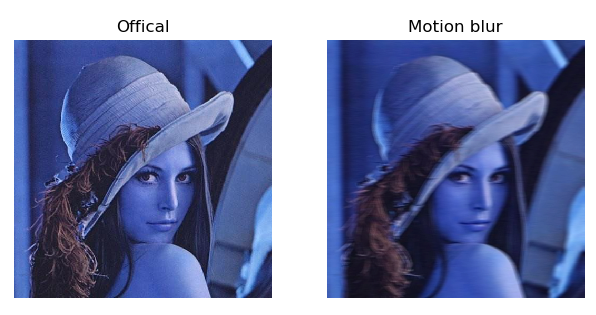展开全文opencv
• 在本文中，我将带您了解图像处理的一些基本功能。特征提取。但是这里我们需要更深入的数据清理。但是数据清理是在数据集，表格，文本等上完成的。如何在图像上完成？ 导入图像python导入图像很容易。以下代码将...
原文链接：http://tecdat.cn/?p=9015

原文出处：拓端数据部落公众号

在本文中，我将带您了解图像处理的一些基本功能。特征提取。但是这里我们需要更深入的数据清理。但是数据清理是在数据集，表格，文本等上完成的。如何在图像上完成？

导入图像

用python导入图像很容易。以下代码将帮助您在Python上导入图像：

image = imread(r"C:\Users\Tavish\Desktop\7.jpg")
show_img(image)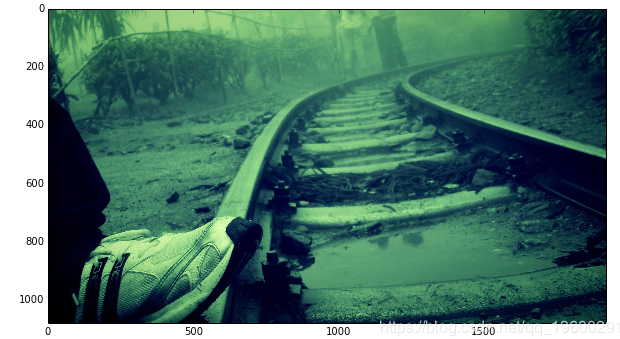了解基础数据

该图像具有多种颜色和许多像素。为了可视化该图像的存储方式， 将每个像素视为矩阵中的一个单元。现在，该单元格包含三种不同的强度信息，分别对应于红色，绿色和蓝色。因此，RGB图像变为3-D矩阵。

red, yellow =   image.copy(), image.copy()
red[:,:,(1,2)] = 0
yellow[:,:,2]=0
show_images(images=[red,yellow], titles=['Red Intensity','Yellow Intensity'])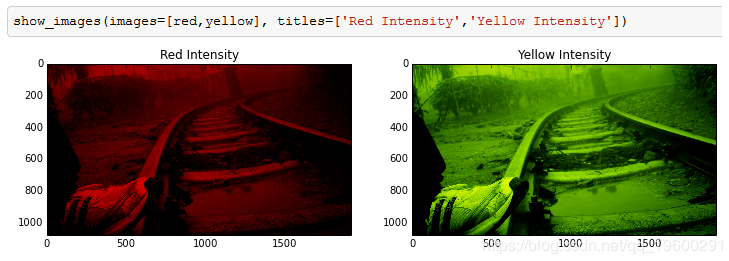将图像转换为二维矩阵

在特征提取中，如果将图像压缩为二维矩阵，则变得更加简单。这是通过灰度或二值化完成的。

这是将RGB图像转换为灰度的方法：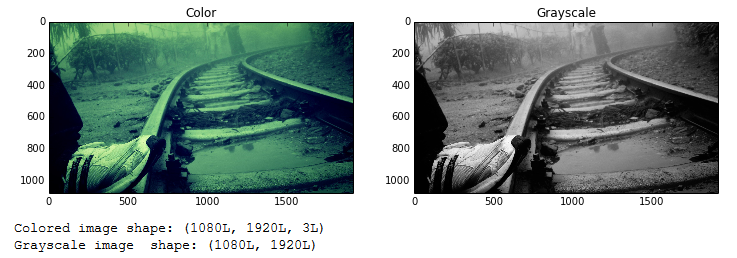现在，让我们尝试对该灰度图像进行二值化处理。这是通过找到阈值并标记灰度像素来完成的。在本文中，我使用了Otsu的方法来找到阈值。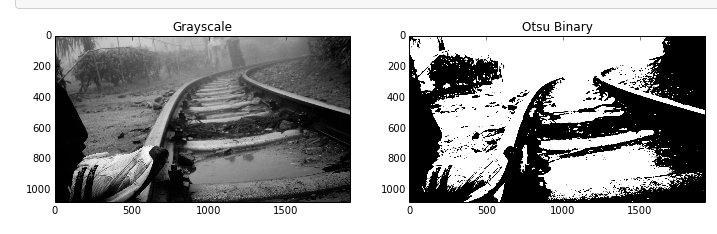模糊影像

我们将在本文中介绍的最后一部分与特征提取更相关：图像模糊。灰度或二进制图像有时会捕获比所需图像更多的图像，在这种情况下模糊处理非常方便。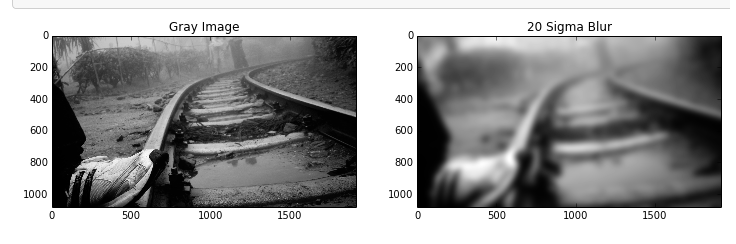在上面的图片中，经过模糊处理后，我们可以清楚地看到鞋子现在已达到与铁路轨道相同的强度水平。因此，该技术在很多图像处理场景中非常方便。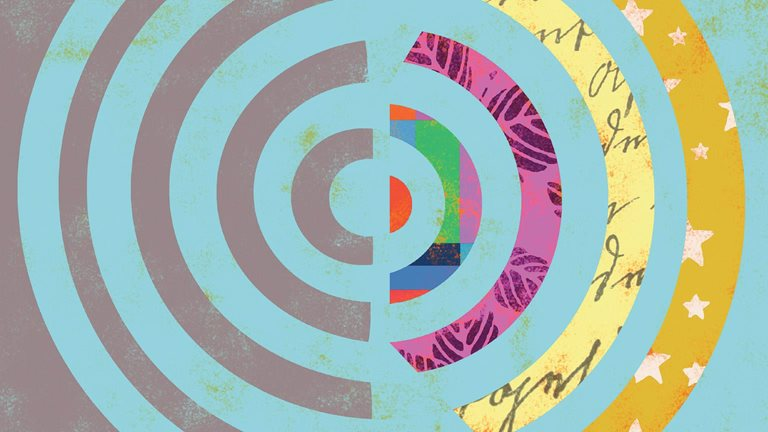最受欢迎的见解

1.使用opencv在python中进行图像处理的简介

2.matlab中的偏最小二乘回归（plsr）和主成分回归（pcr）

3.matlab中使用vmd变分模态分解

4.matlab使用hampel滤波去除异常值

5.matlab使用经验模式分解emd-对信号进行去噪

6.matlab中的偏最小二乘回归（plsr）和主成分回归（pcr）

7.matlab使用copula仿真优化市场风险

8.r语言高级图像处理

9.matlab实现mcmc的马尔可夫切换arma-garch模型估计

展开全文特征提取
• 欢迎关注“小白玩转Python”，发现更多 “有趣”引言在之前的文章中，我们讨论了边缘检测内核。在本文我们将讨论如何在图像上应用模糊与锐化内核，将这些内核应用到彩色图像上，同时保留核心...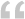欢迎关注 “小白玩转Python”，发现更多 “有趣”引言在之前的文章中，我们讨论了边缘检测内核。在本文我们将讨论如何在图像上应用模糊与锐化内核，将这些内核应用到彩色图像上，同时保留核心图像。一如既往，我们从导入所需的python库开始。import numpy as np
import matplotlib.pyplot as plt
from skimage.io import imshow, imread
from skimage.color import rgb2yuv, rgb2hsv, rgb2gray, yuv2rgb, hsv2rgb
from scipy.signal import convolve2d
读取本文将使用的图像。dog = imread('fire_dog.png')
plt.figure(num=None, figsize=(8, 6), dpi=80)
imshow(dog);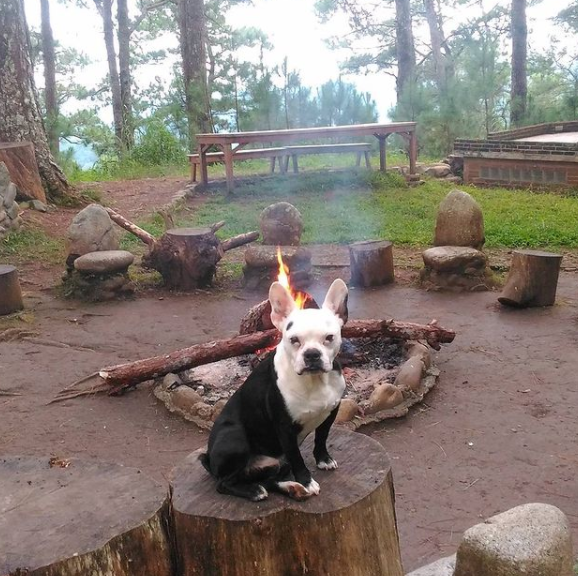现在我们应用于图像的内核是高斯模糊内核和锐化内核。# Sharpen
sharpen = np.array([[0, -1, 0],
[-1, 5, -1],
[0, -1, 0]])
# Gaussian Blur
gaussian = (1 / 16.0) * np.array([[1., 2., 1.],
[2., 4., 2.],
[1., 2., 1.]])
fig, ax = plt.subplots(1,2, figsize = (17,10))
ax.imshow(sharpen, cmap='gray')
ax.set_title(f'Sharpen', fontsize = 18)

ax.imshow(gaussian, cmap='gray')
ax.set_title(f'Gaussian Blur', fontsize = 18)

[axi.set_axis_off() for axi in ax.ravel()];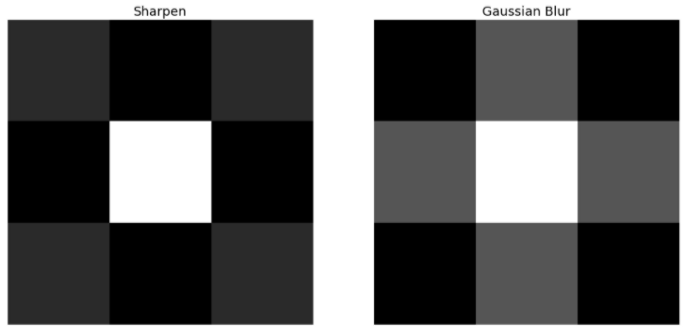但我们如何将这些内核应用到我们的图像中呢？那么，让我们直接通过卷积来试试。def multi_convolver(image, kernel, iterations):
for i in range(iterations):
image = convolve2d(image, kernel, 'same', boundary = 'fill',
fillvalue = 0)
return image
multi_convolver(dog, gaussian, 2)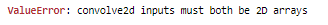很显然，我们出现了维数错误。当我们将一个矩阵与另一个矩阵卷积时，它们的维数应该是相同的。因此，我们必须先将图像转化为灰度。dog_grey = rgb2gray(dog)
plt.figure(num=None, figsize=(8, 6), dpi=80)
imshow(dog_grey);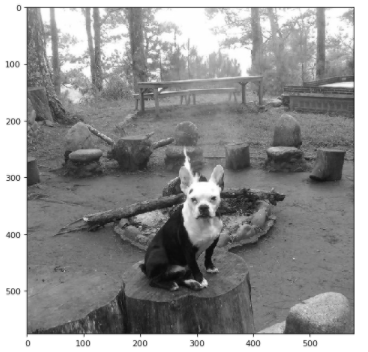现在我们可以运行上面的卷积函数并得到预期的效果。convolved_image = multi_convolver(dog_grey, gaussian, 2)
plt.figure(num=None, figsize=(8, 6), dpi=80)
imshow(convolved_image);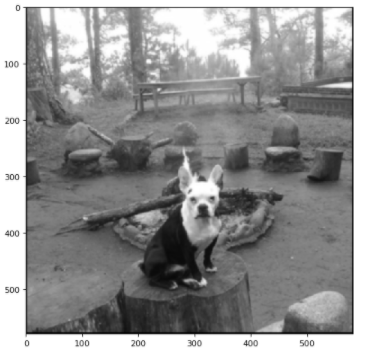我们可以清楚的看到，图像已经模糊了。下面的代码将告诉我们如果继续运行高斯模糊卷积图像会发生什么。def convolution_plotter(image, kernel):
iterations = [1,10,20,30]
f_size = 20

fig, ax = plt.subplots(1,4, figsize = (15,7))
for n, ax in enumerate(ax.flatten()):
ax.set_title(f'Iteration : {iterations[n]}', fontsize =
f_size)
ax.imshow(multi_convolver(image, kernel, iterations[n]),
cmap='gray')
ax.set_axis_off()
fig.tight_layout()

convolution_plotter(dog_grey, gaussian)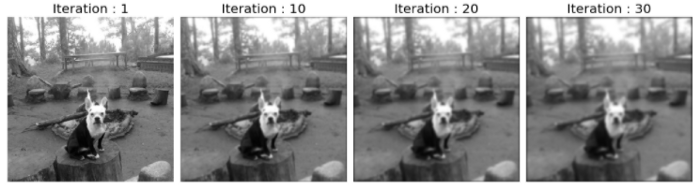可以看到图像变得越来越模糊了。如果需要使图像模糊并保留颜色怎么办？让我们首先尝试对每个颜色通道应用卷积。def convolver_rgb(image, kernel, iterations = 1):
convolved_image_r = multi_convolver(image[:,:,0], kernel,
iterations)
convolved_image_g = multi_convolver(image[:,:,1], kernel,
iterations)
convolved_image_b  = multi_convolver(image[:,:,2], kernel,
iterations)

reformed_image = np.dstack((np.rint(abs(convolved_image_r)),
np.rint(abs(convolved_image_g)),
np.rint(abs(convolved_image_b)))) /
255

fig, ax = plt.subplots(1,3, figsize = (17,10))

ax.imshow(abs(convolved_image_r), cmap='Reds')
ax.set_title(f'Red', fontsize = 15)

ax.imshow(abs(convolved_image_g), cmap='Greens')
ax.set_title(f'Green', fontsize = 15)

ax.imshow(abs(convolved_image_b), cmap='Blues')
ax.set_title(f'Blue', fontsize = 15)

[axi.set_axis_off() for axi in ax.ravel()]

return np.array(reformed_image).astype(np.uint8)
convolved_rgb_gauss = convolver_rgb(dog, gaussian, 2)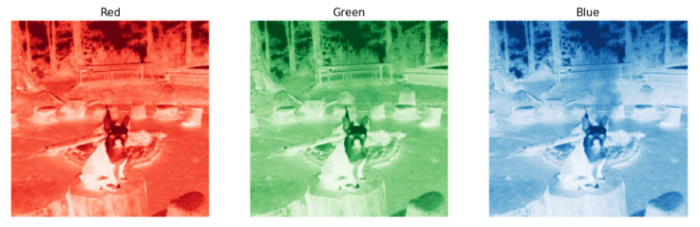上面这个函数实际上将给我们返回重新生成的图像，我们只需要将其插入到imshow函数中即可。plt.figure(num=None, figsize=(8, 6), dpi=80)
imshow(convolved_rgb_gauss);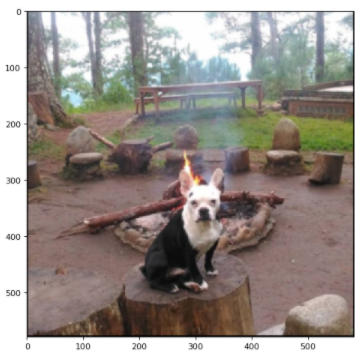让我们看看卷积十次后图像会变成什么样。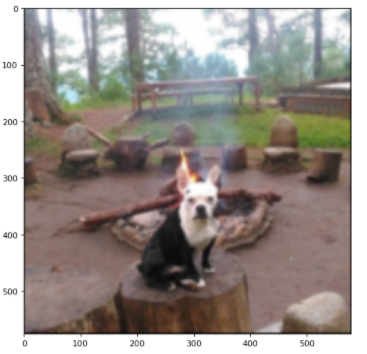现在让我们尝试下更换锐化内核会发生什么。convolved_rgb_sharpen = convolver_rgb(dog, sharpen, 1)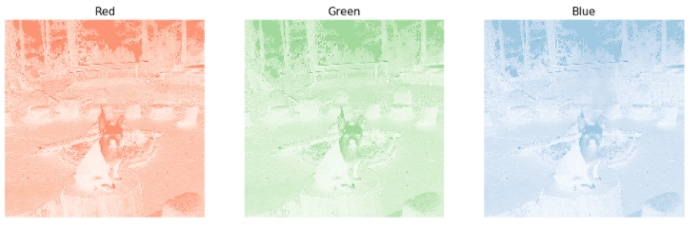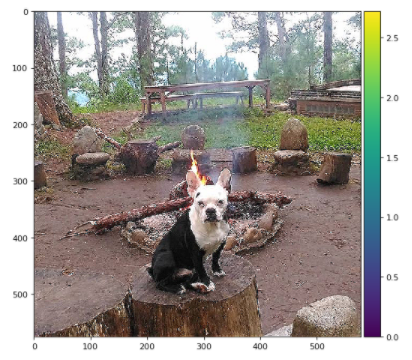处理后的图像有一些轻微的扭曲，为什么会这样呢？请记住，RGB颜色空间隐式地将像素的光照与颜色混合。这意味着在不改变颜色的情况下对图像的光照应用卷积几乎是不可能的。那么我们如何处理这个问题呢？解决此问题的一种方法是更改图像的色彩空间。除了使用RGB颜色空间外，我们还可以使用YUV颜色空间。因为YUV空间中的光照通道实际上是与颜色分开的。现在我们编写一个函数，它首先将图像转换为YUV色彩空间，然后对图像进行卷积。def convolver_rgb(image, kernel, iterations = 1):
img_yuv = rgb2yuv(image)
img_yuv[:,:,0] = multi_convolver(img_yuv[:,:,0], kernel,
iterations)
final_image = yuv2rgb(img_yuv)

fig, ax = plt.subplots(1,2, figsize = (17,10))

ax.imshow(image)
ax.set_title(f'Original', fontsize = 20)

ax.imshow(final_image);
ax.set_title(f'YUV Adjusted, Iterations = {iterations}',
fontsize = 20)

[axi.set_axis_off() for axi in ax.ravel()]

fig.tight_layout()

return final_image
final_image = convolver_rgb(dog, sharpen, iterations = 1)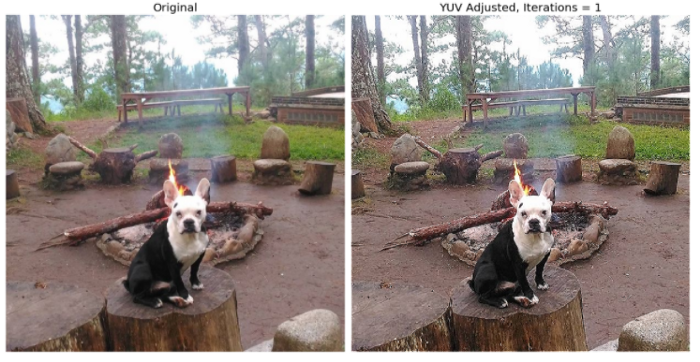现在可以看到，处理后的图像是明显锐化且没有任何颜色扭曲的。还有许多其他的方法来解决这个问题，YUV转换只是其中之一，我们还可以使用HSV来解决这个问题。def convolver_comparison(image, kernel, iterations = 1):
img_yuv = rgb2yuv(image)
img_yuv[:,:,0] = multi_convolver(img_yuv[:,:,0], kernel,
iterations)
final_image_yuv = yuv2rgb(img_yuv)

img_hsv = rgb2hsv(image)
img_hsv[:,:,2] = multi_convolver(img_hsv[:,:,2], kernel,
iterations)
final_image_hsv = hsv2rgb(img_hsv)

convolved_image_r = multi_convolver(image[:,:,0], kernel,
iterations)
convolved_image_g = multi_convolver(image[:,:,1], kernel,
iterations)
convolved_image_b  = multi_convolver(image[:,:,2], kernel,
iterations)

final_image_rgb = np.dstack((np.rint(abs(convolved_image_r)),
np.rint(abs(convolved_image_g)),
np.rint(abs(convolved_image_b)))) /
255

fig, ax = plt.subplots(2,2, figsize = (17,17))

ax.imshow(image)
ax.set_title(f'Original', fontsize = 30)

ax.imshow(final_image_rgb);
ax.set_title(f'RGB Adjusted, Iterations = {iterations}',
fontsize = 30)
fig.tight_layout()

ax.imshow(final_image_yuv)
ax.set_title(f'YUV Adjusted, Iterations = {iterations}',
fontsize = 30)

ax.imshow(final_image_hsv)
ax.set_title(f'HSV Adjusted, Iterations = {iterations}',
fontsize = 30)

[axi.set_axis_off() for axi in ax.ravel()]

fig.tight_layout()
convolver_comparison(dog, sharpen, iterations = 1)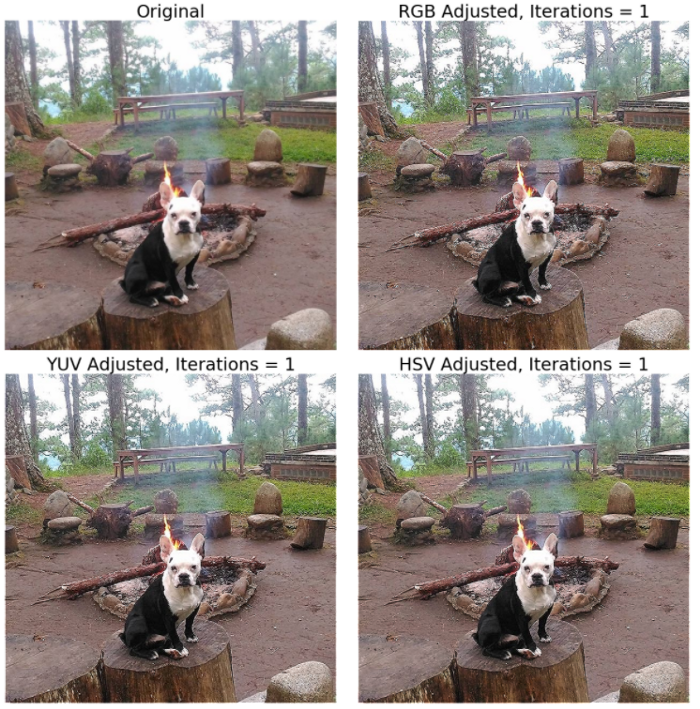我们看到，与原始RGB方法相比，HSV和YUV有了一些改进。为了更好地说明，我们可以将迭代次数从1增加到2。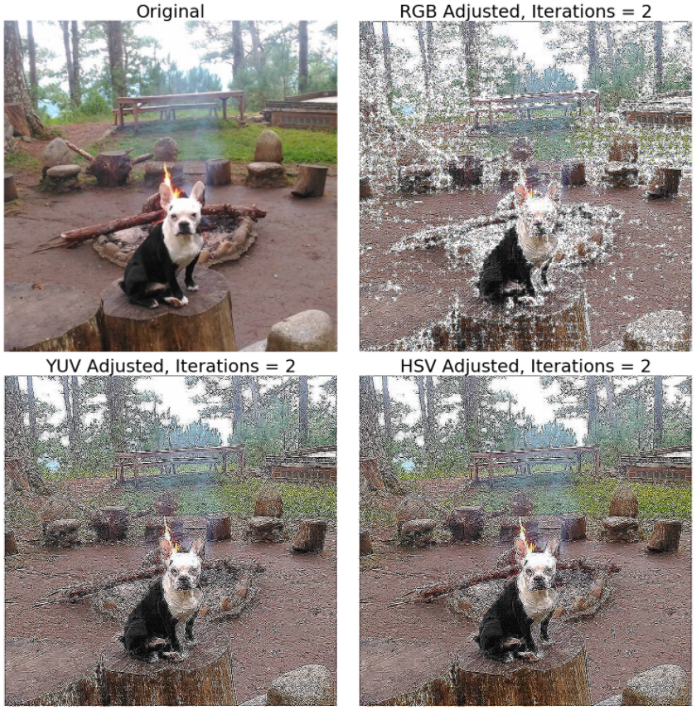在2次迭代后，失真变得更加明显。但是也很清楚，HSV和Y'UV调整后的图像比原始RGB调整后的图像要好得多。在选择将卷积内核应用于图像的最佳方法时，应当牢记这些特性。总结在本文中，我们已经学会了如何对图像进行模糊和锐化卷积。这些技术对于任何从事图像处理和计算机视觉领域的人员都是至关重要的。非常重要的是，我们了解到，简单地将卷积应用到各个RGB通道可能不是最好的方法。在处理图像时，我们应该始终意识到有大量不同类型的色彩空间可以使用。·  END  ·HAPPY LIFE展开全文内核 计算机视觉 opencv 人工智能
• python图像处理：基于canny的动态边缘模糊效果原理canny边缘检测代码 效果 流程如下:先选择canny的两个阈值，确定好需要进行模糊的边缘，再选择模糊程度 原理 canny边缘检测 参考链接 Canny边缘检测算法可以分为...
python图像处理：基于canny的动态边缘模糊效果原理canny边缘检测代码
效果
流程如下:先选择canny的两个阈值，确定好需要进行模糊的边缘，再选择模糊程度原理
canny边缘检测
参考链接
Canny边缘检测算法可以分为以下5个步骤：

使用高斯滤波器，以平滑图像，滤除噪声。

计算图像中每个像素点的梯度强度和方向。

应用非极大值（Non-Maximum Suppression）抑制，以消除边缘检测带来的杂散响应。

应用双阈值（Double-Threshold）检测来确定真实的和潜在的边缘。

通过抑制孤立的弱边缘最终完成边缘检测。

代码
import cv2
t1 = 0
t2 = 0
def Preview(img_path,ts1,ts2):
img = cv2.imread(img_path)
img_copy = img.copy()

imgCanny = cv2.Canny(img,ts1,ts2)

g = cv2.getStructuringElement(cv2.MORPH_RECT, (5, 5))
g2 = cv2.getStructuringElement(cv2.MORPH_RECT, (7, 7))

img_dilate = cv2.dilate(imgCanny, g)
img_dilate2 = cv2.dilate(imgCanny, g2)

shape = img_dilate.shape
for i in range(shape):
for j in range(shape):
if img_dilate2[i,j] == 0:
img[i,j] = [0,0,0]

cv2.namedWindow("Preview",cv2.WINDOW_NORMAL)
cv2.createTrackbar("kerner_size","Preview",0,7,nothing)
while(1):
size = cv2.getTrackbarPos("kerner_size","Preview")
dst = cv2.GaussianBlur(img,(2*size+1,2*size+1),0,0,cv2.BORDER_DEFAULT)

for i in range(shape):
for j in range(shape):
if img_dilate[i,j] != 0:
img_copy[i,j] =dst[i,j]

cv2.imshow("Result",img_copy)
k = cv2.waitKey(1) & 0xff
if k == 27:
break
cv2.destroyAllWindows()

def Choose_Thre(img_path):
global t1,t2
img = cv2.imread(img_path)
gray = cv2.cvtColor(img,cv2.COLOR_BGR2GRAY)

cv2.namedWindow("Preview",cv2.WINDOW_NORMAL)

def nothing(WEV):
pass

cv2.createTrackbar("Threshold1","Preview",0,255,nothing)
cv2.createTrackbar("Threshold2","Preview",0,255,nothing)
while(1):
t1 = cv2.getTrackbarPos("Threshold1","Preview")
t2 = cv2.getTrackbarPos("Threshold2","Preview")
detected_edges = cv2.GaussianBlur(gray,(3,3),0)
detected_edges = cv2.Canny(detected_edges,t1,t2,apertureSize = 3)
dst = cv2.bitwise_and(img,img,mask = detected_edges)
cv2.imshow('Edge',dst)
k = cv2.waitKey(1)&0xFF
if k==27:
break
cv2.destroyAllWindows()

def nothing(wte):
pass

#按esc退出 直接关闭无效
img_path = '1.jpg'
Choose_Thre(img_path)
print(t1,t2)
Preview(img_path,t1,t2)



展开全文算法 opencv 计算机视觉
• 主要介绍了Python scipy的二维图像卷积运算与图像模糊处理操作,涉及Python数学运算与图形绘制相关操作技巧,需要的朋友可以参考下
• 最近在做人脸识别清洗样本的工作，发现经过人脸对齐后存在部分图片...图片越模糊，imageVar的值越小，图像模糊。 #-*-coding:utf-8-*- import sys #reload(sys) #sys.setdefaultencoding('utf-8') import os i...
• 文章目录Pillow模块讲解一、Image模块1.1 、打开图片和显示图片1.2、创建一个简单的图像1.3、图像混合（1...图像旋转（2）格式转换1.5、分离和合并（1）分离（2）合并二、ImageFilter2.1、高斯模糊2.2、其它滤镜三、...
• 由于成像系统、传输介质和记录设备等的不完善，数字图像在其形成、传输记录过程中往往会受到多种噪声的污染...Python中常用Pillow库提供的ImageFilter对图像进行滤镜处理， 包含模糊、平滑、锐化、边界增强等滤镜效...
• 图像处理过程中，会经常涉及”滤波”和”模糊”，两个概念容易混淆，两个概念既有联系又有区别，简单来说，如果将滤波分为低通滤波和高通滤波，低通就是模糊，高通就是锐化。 关于滤波与模糊操作，Opencv中常用函数...opencv 计算机视觉
• python图像处理 学习第一天打卡 图像基础知识 图像都是由像素构成的，像素呢是图像的最小单位，形象的解释想象有一个点阵图，这个点阵图中有许多的小方格，每个小方格都有确切的位置和被分配的色彩，这个小方格就是...opencv
• 计算机视觉–python图像处理基础前言一、图像轮廓和直方图1.1 原理介绍1.2 代码实现及结果二、高斯滤波（高斯模糊）2.1 原理介绍2.2 代码实现及结果三、直方图均衡化3.1 原理介绍3.2 代码实现及结果 前言  图像处理...
• 1、模糊操作：均值模糊、中值模糊、自定义模糊 #均值模糊、中值模糊、自定义模糊 #模糊操作的基本原理 #1、基于离散卷积 #2、定义好每个卷积核 #3、不同卷积核得到不同的卷积效果 #4、模糊是卷积的一种表现 def ...opencv
• PIL-Python图像库1.1 图片的格式转换1.2 创建缩略图1.3拷贝并粘贴区域1.4 调整尺寸及旋转2. Matplotlib库2.1 画图、描点和线2.2 图像轮廓和直方图2.3交互注释3. NumPy库3.1 图像数组表示3.2 灰度变换3.3 直方图均衡...
• 实现图像模糊 关键函数blur(img,size):需要处理的图像，后面的参数为处理的尺寸,size同前面的腐蚀处理一样都是一个n*m的格式 import cv2 as cv img1=cv.imread('5b90f4871daba (1).jpg',1) cv.imshow('origin',img1)...opencv 计算机视觉
• SciPy 提供很多高效的操作，可以实现数值积分、优化、统计... 图像模糊1.图像的高斯模糊是非常经典的图像卷积例子本质上，图像模糊就是将（灰度）图像I 和一个高斯核进行卷积操作： I = I*G 其中* 表示卷积操作。
• 看了许多资料，在图像上个人觉得卷积就是：对于某一位置的像素，通过算法来把它附近的所有像素点的值联合起来，重新设置这个像素的大小。（大概就是这样）这个算法类似有：均值，中值，就是取周围所有像素的均值、...
• ## 教你用Python做图像处理

万次阅读 多人点赞 2018-11-18 15:02:40
提到图像处理第一个想到的库就是PIL，全称Python Imaging Library Python图像处理类库，它提供了大量的图像操作，比如图像缩放，裁剪，贴图，模糊等等，很多时候它需要配合numpy库一起使用 1.open() 你可以使用...
• 图像平滑实际上是低通滤波，平滑过程会导致图像边缘模糊化。 图像平滑是指用于突出图像的宽大区域、低频成分、主干部分或抑制图像噪声和干扰高频成分的图像处理方法，目的是使图像亮度平缓渐变，减小突变梯度，改善...
• 模糊操作是图像处理中最简单和常用的操作之一，该使用的操作之一原因就为了给图像预处理时减低噪声，基于数学的卷积操作 均值模糊，函数 cv2.blur(image,(5,5))，这是一个平滑图片的函数，它将一个区域内所有点的...
• 之前需要评估图像质量来筛选成像质量不错的图片，去除由于对焦，运动等造成的模糊图像，所以在构建数据集的时候考虑用opencv对清晰的图片进行处理获得模糊的图片从而进行训练。 运动模糊图像 一般来说，运动模糊...
• 但是对于很多刚入门的小伙伴来说，如何去灵活应用这些接口着实让人头疼，本文主要就是通过理论与实践相结合的方式，利用 OpenCV-Python 库进行图像处理。 通过本文的学习，你将学到如下知识： 图像预处理阶段 读取...
• opencv-python图像处理（六） 图像的平滑 cv2.blur() 平均模糊，用卷积框内像素的平均值代替卷积框内像素的值 cv2.GaussianBlur() 高斯模糊,常用于去除高斯噪声，中心点邻近区域像素的高斯加权平均值 cv2.medianBlur...opencv 计算机视觉 卷积
• 导入库函数： import chardet import numpy as np import cv2 as cv import cv2 from PIL import Image import sys ...平滑模糊滤波： 均值滤波blur()、 高斯滤波GaussianBlur()、 中值滤波medianB...滤波
• 模糊操作（blur）1.基本概念及API简介2.代码示例3.结果展示 1.基本概念及API简介 均值模糊： cv2.blur(src: Any, ksize: Any, dst: Any = None, anchor: Any = None, borderType: Any = None) 中值模糊： cv2....openCV...

# python图像模糊处理python 订阅